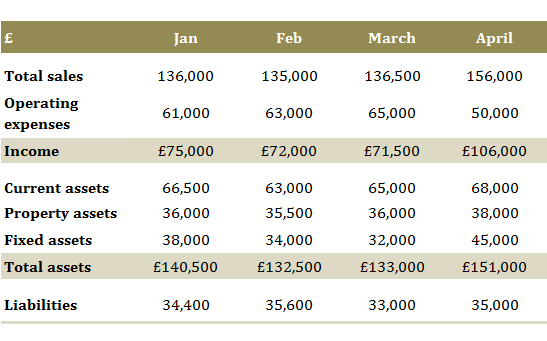# Category Archives: Percentages

## Classic Question 1: “What was the percentage change in current assets from March to April?”Step 1: Locate the right numbers 65,000 and 68,000

Make sure you start with the right numbers from the table, since there are no ‘method marks’ for numerical reasoning tests. Double check that you are looking at the ‘current assets’ row and the March – April columns.

Step 2: Know how to calculate percentage changes

The key here is to know the shortcut method: simply calculate 68,000 ÷ 65,000 = 1.046.

Ans = 4.6% increase.

So how did I know the answer is an increase of 4.6%?

I could have calculated the next step (1.046 – 1) x 100 = 4.6% but from experience I knew that I could just look at the number 1.046 and covnert this into an increase of 4.6% in my head. I know that with this shortcut method a number larger than 1 is an increase, and a number less than 1 is a decrease. The shortcut method is simply a cancelled-down version of the long-hand version:

[ (68,000 – 65,000) ÷ (65,000) ] x 100 = 4.6%

Watch out: the trick to any percentage change question is getting the ‘from’ and ‘to’ number the right way around. The ‘to’ number needs to go on top of the division calculation (the ‘numerator’) and the ‘from’ number needs to go on the bottom (the ‘denominator’). So looking again at our shortcut method we have:

68,000 ÷ 65,000 NOT 65,000 ÷ 68,000.